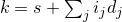# 26 Numerics library [numerics]

## 26.6 Numeric arrays [numarray]

### 26.6.6 The gslice class [class.gslice]

#### 26.6.6.1 The gslice class overview [class.gslice.overview]

namespace std {
class gslice {
public:
gslice();
gslice(size_t s, const valarray<size_t>& l, const valarray<size_t>& d);

size_t           start() const;
valarray<size_t> size() const;
valarray<size_t> stride() const;
};
}


This class represents a generalized slice out of an array. A gslice is defined by a starting offset (s), a set of lengths (lj), and a set of strides (dj). The number of lengths shall equal the number of strides.

A gslice represents a mapping from a set of indices (ij), equal in number to the number of strides, to a single index k. It is useful for building multidimensional array classes using the valarray template, which is one-dimensional. The set of one-dimensional index values specified by a gslice arewhere the multidimensional indices ij range in value from 0 to lij - 1.

Example: The gslice specification

start  = 3
length = {2, 4, 3}
stride = {19, 4, 1}


yields the sequence of one-dimensional indices

k = 3 + (0,1) × 19 + (0,1,2,3) × 4 + (0,1,2) × 1

which are ordered as shown in the following table:

	(i0,   i1,   i2,   k)   =
(0,   0,   0,    3),
(0,   0,   1,    4),
(0,   0,   2,    5),
(0,   1,   0,    7),
(0,   1,   1,    8),
(0,   1,   2,    9),
(0,   2,   0,   11),
(0,   2,   1,   12),
(0,   2,   2,   13),
(0,   3,   0,   15),
(0,   3,   1,   16),
(0,   3,   2,   17),
(1,   0,   0,   22),
(1,   0,   1,   23),
…
(1,   3,   2,   36)


That is, the highest-ordered index turns fastest.  — end example ]

It is possible to have degenerate generalized slices in which an address is repeated.

Example: If the stride parameters in the previous example are changed to {1, 1, 1}, the first few elements of the resulting sequence of indices will be

	(0,   0,   0,    3),
(0,   0,   1,    4),
(0,   0,   2,    5),
(0,   1,   0,    4),
(0,   1,   1,    5),
(0,   1,   2,    6),
…


— end example ]

If a degenerate slice is used as the argument to the non-const version of operator[](const gslice&), the resulting behavior is undefined.

#### 26.6.6.2gslice constructors [gslice.cons]

 gslice(); gslice(size_t start, const valarray<size_t>& lengths, const valarray<size_t>& strides); gslice(const gslice&); 

The default constructor is equivalent to gslice(0, valarray<size_t>(), valarray<size_t>()). The constructor with arguments builds a gslice based on a specification of start, lengths, and strides, as explained in the previous section.

#### 26.6.6.3gslice access functions [gslice.access]

 size_t start() const; valarray<size_t> size() const; valarray<size_t> stride() const; 

Returns: The representation of the start, lengths, or strides specified for the gslice.

Complexity: start() is constant time. size() and stride() are linear in the number of strides.